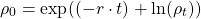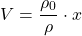# Seeding density

Simply calculate the seeding density you need based on the cells you want to harvest. For a more complex calculation go to seeding calculator.

ρ = density of cell suspension [cells/ cm2]

ρ0= seeding density [cells/ cm2]

ρt = planned harvest density [cells/ cm2]

r = growth rate [1/d]

t = days until next passage

V = volume of suspension per vessel [mL]

x = size of culture vessel [cm2]

N v = number of vessels